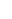# Quiz 11: Performance Measurement in Decentralized Organizations

1)Use the following formula:2)Use the following formula:3)Use the following formula:Calculate residual income: The following steps are involved in calculation of residual income: 1. Multiply the minimum return required with average operating asset. 2. Deduct above calculated amount from net operating income. The following information is given: Net operating income = \$400,000 Average operating assets = \$ 2,200,000 Minimum rate of return = 16% Step 1:Step 2: Residual income is calculated as follows: